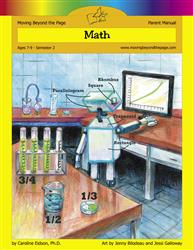# Common Core Alignment

CCSS.Math.Content.3.MD.7.b - Multiply side lengths to find areas of rectangles with whole- number side lengths in the context of solving real world and mathematical problems, and represent whole-number products as rectangular areas in mathematical reasoning.

## 5: MathUnit 5: Area and Perimeter
Lesson 10: Problem Solving With Perimeter and Area
Lesson 11: Unit Test
Lesson 7: Calculating Area
Lesson 8: More Work With Area
Lesson 9: Creating With Perimeter and Area
Final Project: Shapesville